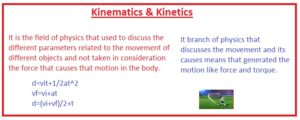Hello, friends welcome to the new post. In this post, we will have a detailed look at Difference Between Kinematics & Kinetics. These 2 terms are used in different bodies in motion states. The kinematics explains the discussion about the facts that different forces in results the movement of any objects.

While kinetics deals with the forces acting on the body that caused-motion in other words kinematics deals with motion and kinetics about forces that caused motion. In this tutorial, we cover the basics of these two parameters. So let’s get started.

## Difference Between Kinematics & Kinetics

Kinematics

• It is the field of physics that used to discuss the different parameters related to the movement of different objects and not taken in consideration the force that causes that motion in the body.
• As it discusses motion so it sometimes considers as motion geometry and also as math field as nature.
• It also helps to study the different parameters related to the velocity and acceleration of anybody.
• Through finding the values of velocity and acceleration we can determine the other parameters related to moving body like position,
• Kinematics not discusses the different physical aspects but also covers the motion of celestial objects and gives an overview of the components of astrophysics.
• The robot’s mechanical engineering and biomedicines’ different parameters related to motion and movement of different parts of machines automobiles are discussed and designed according to different parameters.
• The function of robots also  works in this field of study
• Ther are main four types of kinematics equation that discuss different parameters

d=vit+1/2at^2
vf=vi+at
d=(vi+vf)/2+t### Kinetics

• It branch of physics that discusses the movement and its causes means that generated the motion like force and torque.
• Torque and friction are practical examples of kinetics
• The projectile motion also discuss through this phenomenon
• the energy transformation also discuss through this process
• The term dynamics is used for this field
• Like the kinematics, there is no equation for this field
• The types of kinetics that discuss the motion fo Human and their physical parts are called human kinetics
• It also works in different fields of science like chemistry, bio.
• the main component of the object during the study of this field is mass over which force is applied. In simple words, mass is the main element to discuss the kinetics
• The design of any vehicle like the truck car is created through under consideration this field since motion all parts rely on it

That is a detailed post-bout difference between kinematics and kinetics if you have any further query ask in the comments. Thanks for reading have a nice day.